## Visualize Bode Response of Simulink Model During Simulation

This example shows how to visualize linear system characteristics of a nonlinear Simulink® model during simulation, computed at the model operating point (simulation snapshot time of 0).

For example:

`open_system('watertank')`
2. Open the Simulink Library Browser. In the Simulink Editor, on the Simulation tab, click Library Browser.

1. In the Simulink Control Design library, select Linear Analysis Plots.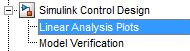2. Drag and drop a block, such as the Bode Plot block, into the model window.

The model now resembles the following figure.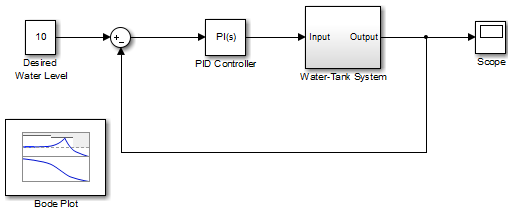4. Double-click the block to open the Block Parameters dialog box.5. Specify the linearization I/O points.

The linear system is computed for the Water-Tank System.

Tip

If your model already contains I/O points, the block automatically detects these points and displays them. Clickat any time to update the Linearization inputs/outputs table with I/Os from the model.

1. To specify an input:

1. Click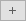adjacent to the Linearization inputs/outputs table.

The Block Parameters dialog expands to display a Click a signal in the model to select it area.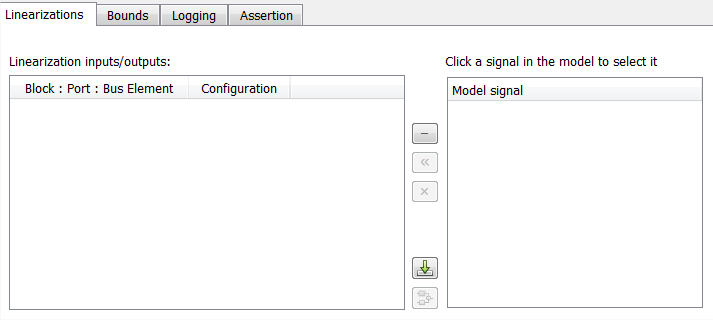Tip

You can select multiple signals at once in the Simulink model. All selected signals appear in the Click a signal in the model to select it area.

2. In the Simulink model, click the output signal of the `PID Controller` block to select it.

The Click a signal in the model to select it area updates to display the selected signal.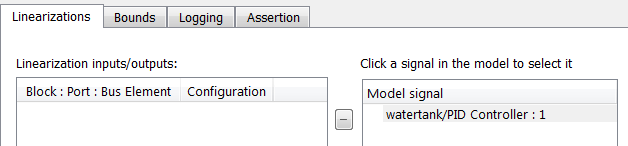3. Click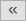to add the signal to the Linearization inputs/outputs table.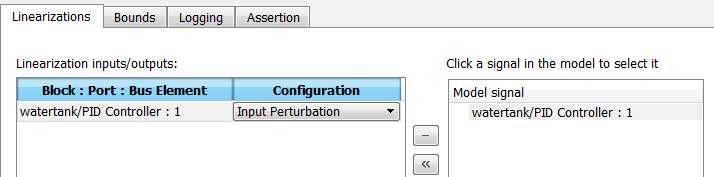2. To specify an output:

1. In the Simulink model, click the output signal of the `Water-Tank System` block to select it.

The Click a signal in the model to select it area updates to display the selected signal.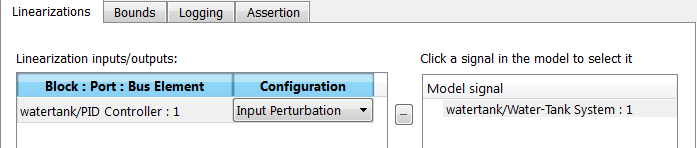2. Clickto add the signal to the Linearization inputs/outputs table.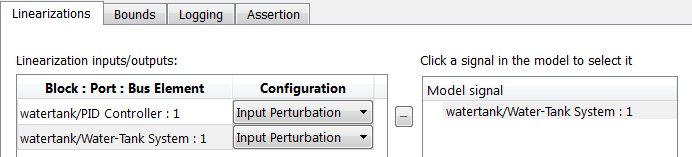3. In the Configuration drop-down list of the Linearization inputs/outputs table, select `Open-loop Output` for watertank/Water-Tank System : 1.

The Linearization inputs/outputs table now resembles the following figure.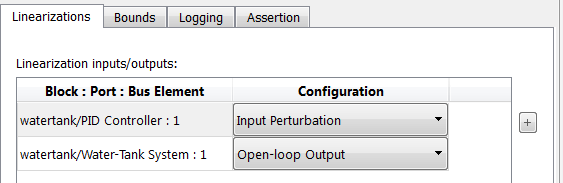3. Click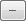to collapse the Click a signal in the model to select it area.

Tip

Alternatively, before you add the Linear Analysis Plots block, right-click the signals in the Simulink model and select Linear Analysis Points > Input Perturbation and Linear Analysis Points > Open-loop Output. Linearization I/O annotations appear in the model and the selected signals appear in the Linearization inputs/outputs table.

6. Save the linear system.

1. Select the Logging tab.

2. Select the Save data to workspace option, and specify a variable name in the Variable name field.

The Logging tab now resembles the following figure.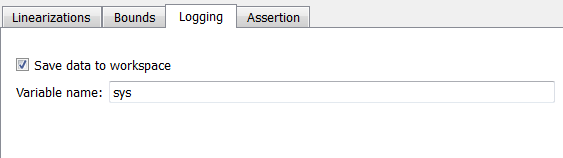7. Click to open an empty plot.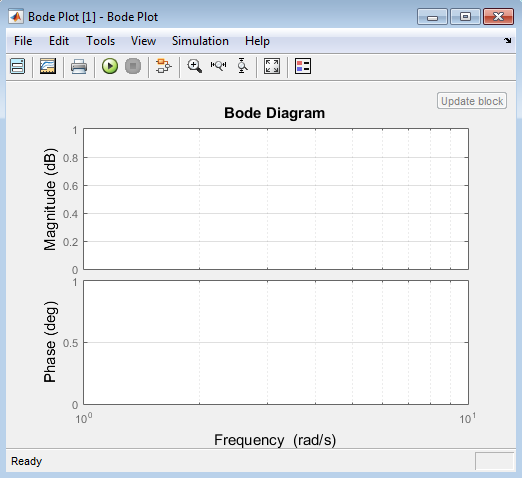8. Plot the linear system characteristics by clicking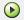in the plot window.

Alternatively, you can simulate the model from the model window.

The software linearizes the portion of the model between the linearization input and output at the default simulation time of 0, specified in Snapshot times parameter in the Block Parameters dialog box, and plots the Bode magnitude and phase.

After the simulation completes, the plot window resembles the following figure.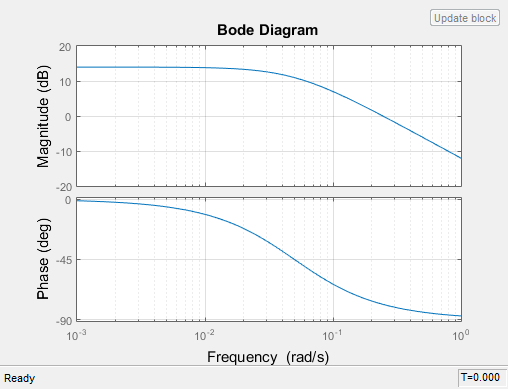The computed linear system is saved as `sys` in the MATLAB® workspace. `sys` is a structure with `time` and `values` fields. To view the structure, type:

`sys`

This command returns the following results:

```sys = time: 0 values: [1x1 ss] blockName: 'watertank/Bode Plot'```
• The `time` field contains the default simulation time at which the linear system is computed.

• The `values` field is a state-space object which stores the linear system computed at simulation time of 0. To learn more about the properties of state-space objects, see `ss`.

(If the Simulink model is configured to save simulation output as a single object, the data structure `sys` is a field in the `Simulink.SimulationOutput` object that contains the logged simulation data. For more information about data logging in Simulink, see Save Simulation Data and the `Simulink.SimulationOutput` reference page.)